Question

A flywheel turns through 26 rev as it slows from an angular speed of 8.5 rad/s to a stop. (a) Assuming a constant angular acceleration, find the time for it to come to rest. (b) What is its angular acceleration? (c) How much time is required for it to complete the first 13 of the 26 revolutions?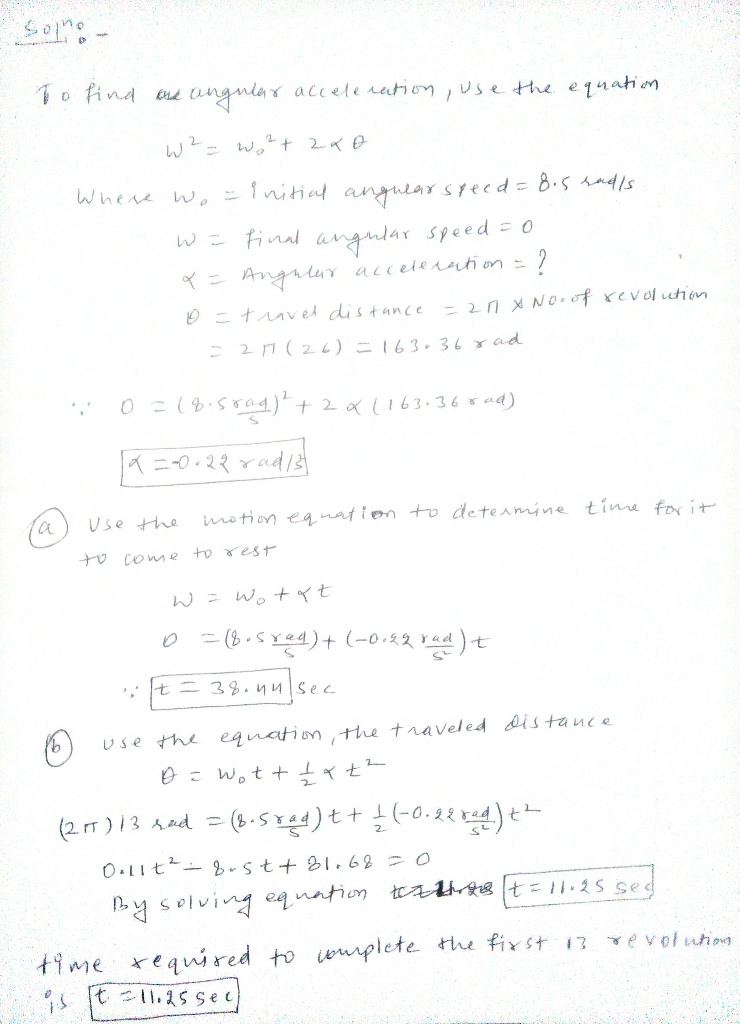#### Earn Coins

Coins can be redeemed for fabulous gifts.

Similar Homework Help Questions
• ### A flywheel turns through 28 rev as it slows from an angular speed of 8.9 rad/s...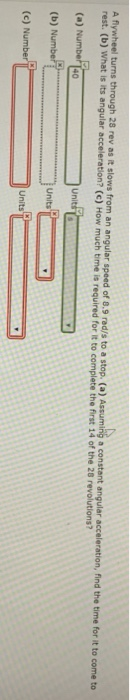A flywheel turns through 28 rev as it slows from an angular speed of 8.9 rad/s to a stop. (a) Assuming a constant angular acceleration, find the time for it to come to rest. (b) What is its angular acceleration? (c) How much time is required for it to complete the first 14 of the 26 revolutions? (a) Number 140 (b) Number (c) Number unt: U Units

• ### A flywheel turns through 22 rev as it slows from an angular speed of 5.6 rad/s...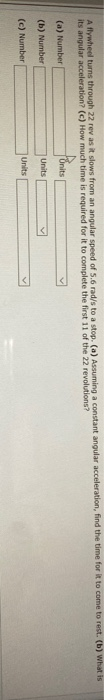A flywheel turns through 22 rev as it slows from an angular speed of 5.6 rad/s to a stop. (a) Assuming a constant angular acceleration, find the time for it to come to rest. (b) What is its angular acceleration? (c) How much time is required for it to complete the first 11 of the 22 revolutions? Units (a) Number (b) Number (c) Number Units Units

• ### A56 (9.2) A flywheel rotates at a constant angular acceleration of 0.35 rad/s2. It has an...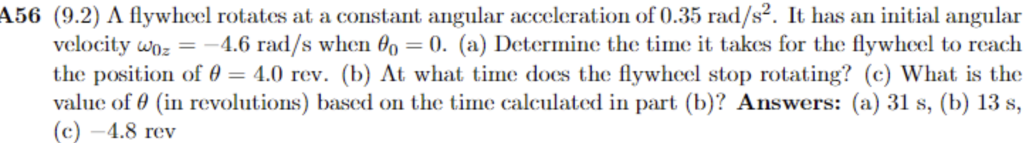A56 (9.2) A flywheel rotates at a constant angular acceleration of 0.35 rad/s2. It has an initial angular velocity uOz 4.6 rad/s when θ(,-0. (a) Detormine the time it takes for the flywheel to reach the position of 4.0 rev. (b) At what time does the flywheel stop rotating? (c) What is the value of θ (in revolutions) based on the time calculated in part (b)? Answers: (a) 31 s, (b) 13 s, c)-4.8 rev

• ### A flywheel with a diameter of 1.60 m is rotating at an angular speed of 320...

A flywheel with a diameter of 1.60 m is rotating at an angular speed of 320 rev/min. (a) What is the angular speed of the flywheel in rad/s? rad/s (b) What is the linear speed of a point on the rim of the flywheel? m/s (c) What constant angular acceleration (in revolutions per minute-squared) will increase the wheel's angular speed to 1000 rev/min in 60 s? rev/min2 (d) How many revolutions does the wheel make during this 60 s acceleration?...

• ### 28 A certain wheel turns through 100 rev in 15 s its angular speed at the...

28 A certain wheel turns through 100 rev in 15 s its angular speed at the end of the period being 12 rev/s (a) What was the angular speed of the wheel at the beginning of the 15-s interval, assuming constant angular acceleration? (b) How much time had elapsed between the time the wheel was at rest and the beginning of the 15-s interval? 29 A hoop of radius 2.5m has a mass if 150 kg. It rolls along a...

• ### A flywheel with a diameter of 0.679 m is rotating at an angular speed of 221...

A flywheel with a diameter of 0.679 m is rotating at an angular speed of 221 rev/min. (a) What is the angular speed of the flywheel in radians per second? (b) What is the linear speed of a point on the rim of the flywheel? (c) What constant angular acceleration (in revolutions per minute-squared) will increase the wheel's angular speed to 730 rev/min in 68.5 s? (d) How many revolutions does the wheel make during that 68.5 s? (a is...

• ### The angular velocity of flywheel decreases uniformly from 12000rev/min to 3000 rev/min in 8 sec. Find...

The angular velocity of flywheel decreases uniformly from 12000rev/min to 3000 rev/min in 8 sec. Find the angular acceleration and the number of revolutions made by the wheel in the 8 sec interval. How many more seconds are required for the wheel to come to rest?

• ### There is a disk rotating with a constant angular speed of 18 rad/sec. At some instant...

There is a disk rotating with a constant angular speed of 18 rad/sec. At some instant the disk begins to experience a constant friction resulting in an angular acceleration of 1.4 rad/sec2 Find: 1) the time (in sec) it takes the disk to come to a complete stop due to friction 2) total number of complete revolutions the disk undergoes before coming to a complete stop due to friction; 3) the time (in sec) it takes the disk to complete...

• ### The flywheel of a steam engine runs with a constant angular speed of 416 rev/min (in...

The flywheel of a steam engine runs with a constant angular speed of 416 rev/min (in the counterclockwise direction). When the steam is shut off, the friction of the bearings stops the wheel in 2.1 h. (HINT: Be careful with the units.) (a) What is the magnitude of the constant angular acceleration, in revolutions per minute-squared, of the wheel during the slowdown? (b) How many revolutions does the wheel make before stopping? (c) At the instant the flywheel is turning...

• ### Problem #4 A disk is rotating with a constant angular speed of 18 rad/sec. At some...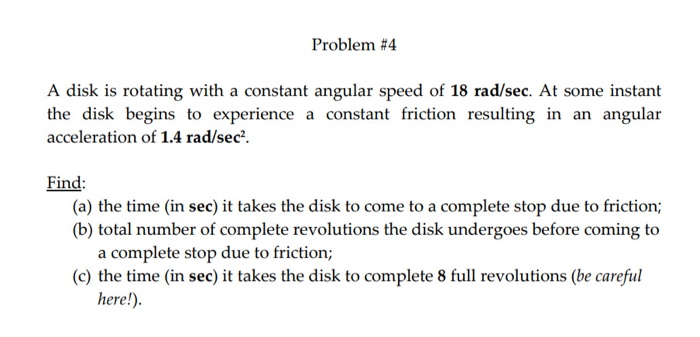Problem #4 A disk is rotating with a constant angular speed of 18 rad/sec. At some instant the disk begins to experience a constant friction resulting in an angular acceleration of 1.4 rad/sec?. Find: (a) the time (in sec) it takes the disk to come to a complete stop due to friction; (b) total number of complete revolutions the disk undergoes before coming to a complete stop due to friction; (c) the time in sec) it takes the disk to...